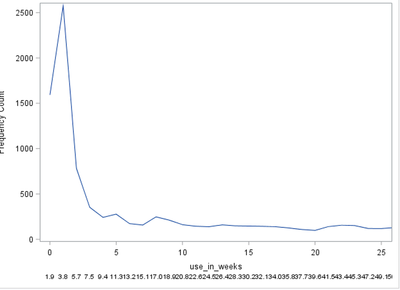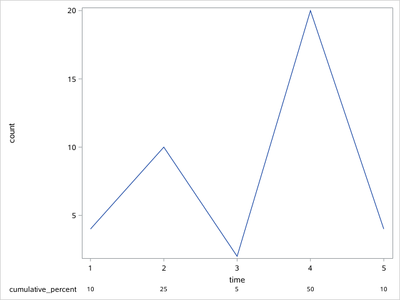## xaxistable

Hello all,

i am creating a really simple sgplot, where i want to use an xaxistable. My problem is, that the xaxistable is not aligned with the x-axis. It shows too many observations.

How can i get the values to align with the values on the x-axis?

example data:

data have;

input time count cumulative_percent;

datalines;

1 4 10

2 10 25

3 2 5

4 20 50

5 4 10

;

run;

``````proc sgplot data = abc;
series x=time y = count;
taxis values = 0 to 52 by 5);
xaxistable cumulative_percent;run;``````1 ACCEPTED SOLUTION

Accepted Solutions

## Re: xaxistable

You might need to create a temporary dataset where those unwanted values are set to missing.

Example:

``````/* Create sample data for demonstration */

data have;
do time=0 to 52;
count=round(pdf('chisq',time,3)*1e4+108.9);
cumulative_percent+count/150;
output;
end;
label time='use_in_weeks';
run;

/* Restrict values for x-axis table to tick marks */

data want;
set have;
if mod(time,5) then cumulative_percent=.;
format cumulative_percent 5.1;
run;

/* Create the plot with blanks between the x-axis table items */

options missing=' ';
proc sgplot data = want;
series x=time y = count;
xaxis values = (0 to 52 by 5);
xaxistable cumulative_percent / label='cumul. %';
run;``````
3 REPLIES 3Ksharp
Super User

## Re: xaxistable

```data have;
input time count cumulative_percent;
datalines;
1 4 10
2 10 25
3 2 5
4 20 50
5 4 10
;
run;

proc sgplot data = have;
series x=time y = count;
*xaxis values =( 0 to 52 by 5);
xaxistable cumulative_percent/x=time;
run;
```## Re: xaxistable

When i add the / x=time to my code, i still have way to many datapoints at my graph.
I have calculated the cumulative percent for each observation, but on my x-axis i have chosen only to show by 5. Can i get the xaxistable to only show by 5 as well?

## Re: xaxistable

You might need to create a temporary dataset where those unwanted values are set to missing.

Example:

``````/* Create sample data for demonstration */

data have;
do time=0 to 52;
count=round(pdf('chisq',time,3)*1e4+108.9);
cumulative_percent+count/150;
output;
end;
label time='use_in_weeks';
run;

/* Restrict values for x-axis table to tick marks */

data want;
set have;
if mod(time,5) then cumulative_percent=.;
format cumulative_percent 5.1;
run;

/* Create the plot with blanks between the x-axis table items */

options missing=' ';
proc sgplot data = want;
series x=time y = count;
xaxis values = (0 to 52 by 5);
xaxistable cumulative_percent / label='cumul. %';
run;``````
Discussion stats
• 3 replies
• 295 views
• 0 likes
• 3 in conversation